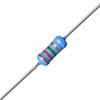# Resistor Fundamentals

## What Is a Resistor?Resistors are passive electrical components that reduce the flow of electrical current in a circuit. They are one of the most common components and can be found in almost every electrical network and electronic circuit. The resistance is expressed in ohms (Ω). There are many different resistor types and constructions. They applications for resistors vary widely: generating heat, limiting current and creating a voltage drop are only a few examples.

## Ohm's Law

Ohm’s law is one of the most elementary equations used in electrical engineering. The law was discovered by Georg Ohm in 1827, and it describes the relation between voltage, current and resistance. When a current passes through a resistor, the voltage drop across the terminals is proportional to the magnitude of resistance:

$$V = I · R$$

## Resistance of a Resistor

The resistance of a resistor is its primary parameter. Resistance is expressed in ohms (Ω) and is dependent on the shape of the resistive part and the material properties. The longer the path the current has to travel across the resistor, the higher the resistance will be. The extent to which a material is resistant to electric current is called resistivity. The symbol for resistivity is ρ and the units are Ω·m. The higher the resistivity of a material, the higher the resistance will be. Good resistor materials have resistivity’s between 2•10-8 and 200•10-8 Ω·m.

## Kirchhoff's Laws

The Kirchhoff circuit laws form the basis of electrical network theory. They are based on the principle of conservation of electrical charge and energy. Kirchhoff's Current Law (KCL), or first law, states that the sum of currents from one node is zero. Kirchhoff's Voltage Law (KVL), or second law, states the sum of voltage drops and rises in a closed loop of a network is zero. Together with Ohm’s law, the Kirchhoff laws make it possible to solve complex electrical networks.

## Resistor Properties

To specify a resistor, the most important parameter is resistance. But dependent on the application, many other properties may play a role. A few examples are given:

### Resistor noise

For some situations, such has high gain amplifiers in audio applications, resistor noise is an unwanted phenomenon. Some resistors have better noise properties than others. Metal foil is among the best, while carbon composition possesses the worst noise properties.

### Temperature coefficient of resistance (TCR)

The relation between resistance and temperature is defined as the temperature coefficient of resistance (TCR), which is measured in ppm/°C or ppm/K. It is primarily dependent on the resistive material properties, but also on the construction of the resistor.

### Power rating

The power rating in watts (W) of a resistor is a measure of the maximum energy a resistor can dissipate without damaging or altering the properties. Based on the operating conditions and environment, the nominal power rating can decrease. For example, at high ambient temperatures, the resistor power rating is de-rated to a lower value. This is expressed in a derating chart.

## Resistor Capacitance and Inductance

An ideal resistor has only resistance and no inductive or capacitive properties. Practical resistors have some series inductance and parallel capacitance. For high frequency circuits these parasitic effects can cause problems. Wirewound resistors are particularly known for their bad high frequency properties.